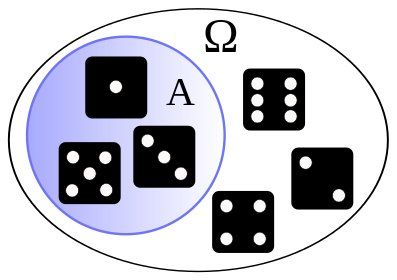# Quantum mechanics and free probabilityEU-funded research has made important progress in deriving mathematical descriptions of quantum probability, an extension of classical probability that has important applications in quantum physics and telecommunication.

The main focus of probability theory is the algebra of random variables. In the conventional approach, one selects a sample space and assigns a probability (expectation) to a number of events in that space, building algebras of random variables. The random variables, the probability of a certain event in that sample space, are commutable, meaning that changing the order of operands does not change the result.

In quantum mechanics, the sample space is replaced by the space of states and the expectation is now the expected value on a given quantum state. Physical observables take the place of the random variables that generally do not commute. Quantum probability, also called noncommutative probability, incorporates the possibility of noncommutative operations, encompassing both the quantum and classical states. Developed in the 1980s, it has provided models of quantum observation processes that resolve many of the apparent inconsistencies of quantum mechanics.

Quantum probability contains many different notions of independence, the most prominent of which is free probability, a concept created around 1985. The discovery in 1991 that it is closely related to random matrix theory led to exciting new results, concepts and tools, and the identification of important applications. The EU-funded project 'Independence and convolutions in noncommutative probability' (ICNCP) investigated the mathematical theory of free probability and free independence, pushing the frontiers of both classical and free probability.

The short two-year research effort resulted in nine publications and 10 presentations. Outcomes will make an important contribution to the field and eventually to the description and development of practical devices.

last modification: 2015-02-27 15:13:10